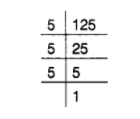# The least number by which 125 be

Question:

The least number by which 125 be multiplied to make it a perfect square, is________

Solution:

5

Resolving 125 into prime factors, we get

$125=5 \times 5 \times 5$

Grouping the factors in triplets of equal factors, we get

$125=(5 \times 5) \times 5$We observe that 5 is without a pair.

Thus, we must multiply the number by 5 , so that the product is a perfect square.

Hence, the least number, which should be multiplied with 125 to make it a perfect square, is 5 .